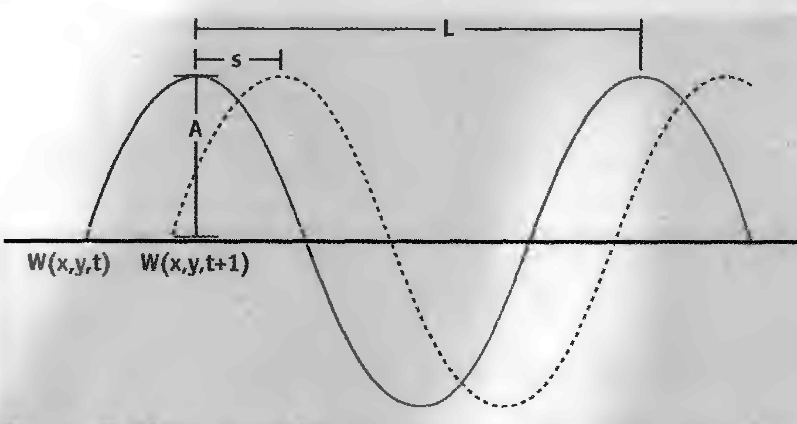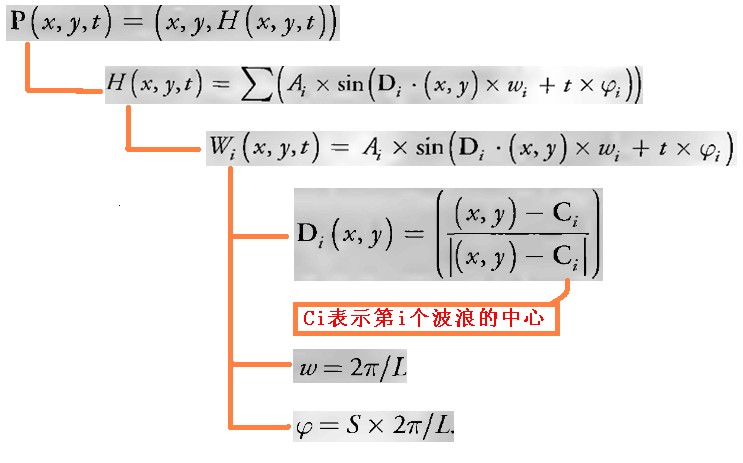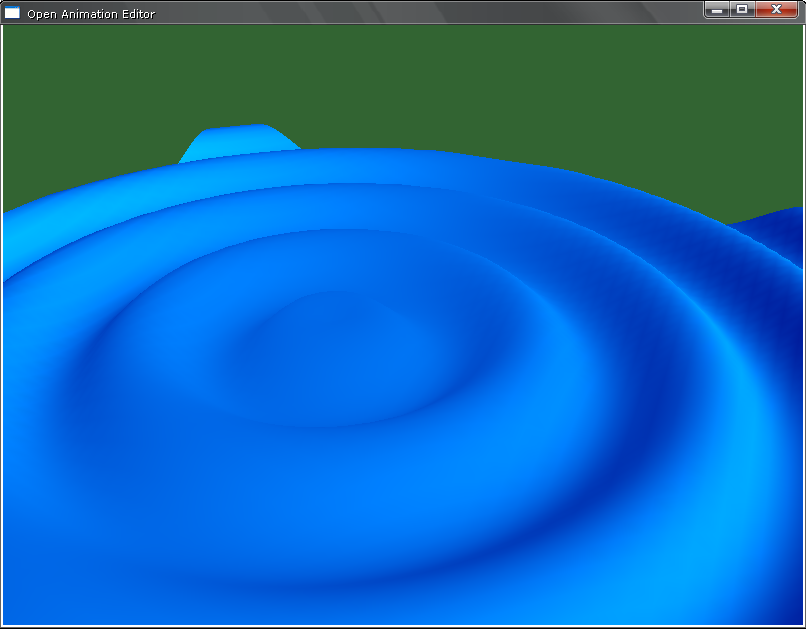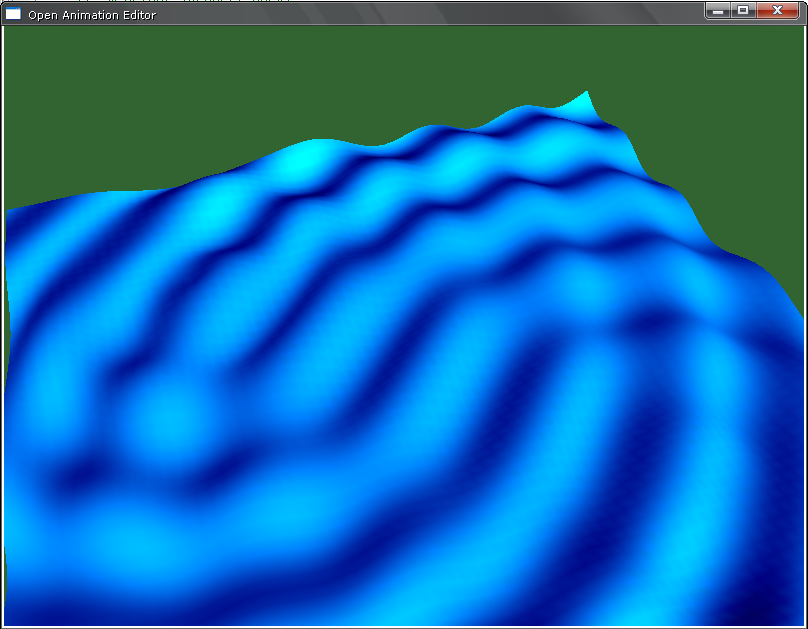最近在研究波浪的制作，但在网上很难找到一些详细地介绍如何制作3D波浪的文章。后来找到了NVIDIA 的一本书《GPU Gems》，刚好在第一章就讲到了如何制作波浪，所用到的算法是 Fast Fourier Transform (FFT)。下面简单地对它作一个解读。
先来想以下波浪是怎么形成的。
在海洋中，大多数波浪是由风产生的。急速流动的空气推动一些水分子聚集起来，掀起汹涌的浪花——海面特定位点的扰动。这些分子推动相邻的分子，相邻的那些分子进而又推动与其相邻的另一些分子，就这样推动下去。通过这种方式，扰动沿着海面传播开来，而单个水分子却大致停留在同一区域。
从波浪的横截面上看，很像正弦曲线，不同之处在于：正弦曲线波峰和波谷的弧度都一样；而波浪的波峰比较尖，波谷比较平滑。下面来研究这个波浪的计算公式。先做以下约定：

L : 一个周期的波浪长度

w: 频率；（ w = 2 *п / L

A: 幅度

S: 速度

φ: 相位（φ= S * 2 *φ / L

D: 波矢量

W(x,y,t) : t 时刻，处于 xy）位置的点受到一个波浪中心的影响而形成的高度

H(x,y,t): t 时刻，处于 xy）位置的点受到所有波浪中心的影响而形成的高度（该点的实际高度）

P(x, y,t): t 时刻，顶点的实际坐标

在这里或者还要说明一下，海上的波浪可能是由多个不同中心的波浪共同作用而形成的，而W(x,y,t) 求的是一个波浪的影响，H(x,y,t)求的是所有波浪的影响。
研究某一点所受到的影响主要有两个，一个是计算某一时刻某一点的实际位置；另外一个是计算某一时刻某一点的法向量。下面先来研究如何计算某一时刻某一点的实际位置。我们可以设计一个水体类 GWater, 一个波浪类 GWave 。GWater 负责计算某一点的实际位置（即受到所有波浪影响后的实际位置）。GWave 负责记录的信息有：

L : 一个周期的波浪长度

w: 频率；（ w = 2 *п / L

A: 幅度

S: 速度

φ: 相位（φ= S * 2 *φ / L

而
D （波矢量）的计算还要涉及到具体的某个点的x和z坐标分量。
一个GWater 里面可以有一个或多个GWave对象。

我做了一个简单的实现，下面是运行效果：

1、一个波浪中心：2、两个波浪中心：GWater.h

//************************************************************

//说明：生成水体

//作者：何家勉

//创建日期：年月日

//修改日期：年月日

//************************************************************

#ifndef _GWater_H_

#define _GWater_H_

#include "GObject.h"

namespace OpenAE

{

//------------------------------------------------------------

//说明：波浪类

//------------------------------------------------------------

class GWave

{

private:

float         m_fSpeed; //速度

float         m_fSwing; //振幅A

float         m_fCycleLength; //一个周期的长度

D3DXVECTOR3       m_vCenter; //

//------------- 下面变量可以由上面的变量求得，

//因此只起到减少重复计算的作用--------------

float         m_fFrequency; //频率

float         m_fPhase; //相位

float         m_fAlpha; // sin( m_fAlpha)

public:

GWave(){};

~GWave(){};

//------------------------------------------------------------

//desc: 构造函数

//param: fSpeed 速度

//param: fSwing 幅度

//param: fCycleLength 一个周期的波长

//param: vCenter 波浪的中心

//------------------------------------------------------------

GWave( float fSpeed, float fSwing, D3DXVECTOR3 vCenter)

{

m_fSpeed = fSpeed;

m_fSwing = fSwing;

m_fCycleLength = fSpeed * 2.0f * D3DX_PI;

m_vCenter = vCenter;

}

//------------------------------------------------------------

//desc: 获取波浪中心到某点的向量的值

//param: staticX 点的x分量

//param: statixZ 点的z分量

//return: 向量的值

//------------------------------------------------------------

float GetDirectionValue(float staticX, float staticZ)

{

float fSign; //决定正负号

//fSign = ( ( staticZ - m_vCenter.z ) >= 0 ) ? 1.0f : -1.0f;

fSign = -1.0f;

float fXLen = ( staticX - m_vCenter.x) * ( staticX - m_vCenter.x);

float fZLen = ( staticZ - m_vCenter.z) * ( staticZ - m_vCenter.z);

float fValue = sqrt( fXLen + fZLen) * fSign;

return fValue;

}

//------------------------------------------------------------

//desc: 获取波浪中心到某点的向量的x分量值

//param: staticX 点的x分量

//return: 向量的x分量值

//------------------------------------------------------------

float GetDirectionX(float staticX){ return staticX - m_vCenter.x;}

//------------------------------------------------------------

//desc: 获取波浪中心到某点的向量的z分量值

//param: statixZ 点的z分量

//return: 向量的z分量值

//------------------------------------------------------------

float GetDirectionZ(float staticZ){ return staticZ - m_vCenter.z;}

//------------------------------------------------------------

//desc: 以下是一系列Get Set操作

//------------------------------------------------------------

void   SetSpeed( float fSpeed){ m_fSpeed = fSpeed;}

float GetSpeed(){ return m_fSpeed;}

void   SetSwing( float fSwing){ m_fSwing = fSwing;}

float GetSwing(){ return m_fSwing;}

void   SetCycleLength(float fLength){ m_fCycleLength = fLength;}

float GetCycleLength(){ return m_fCycleLength;}

void   SetCenter( D3DXVECTOR3 vCenter){ m_vCenter = vCenter;}

D3DXVECTOR3 GetCenter(){ return m_vCenter;}

float GetFrequency(){ return m_fFrequency;}

float GetPhase(){ return m_fPhase;}

//------------------------------------------------------------

//desc: 在每次循环调用次类时都要先调用此方法，用于初始化某些变量

//------------------------------------------------------------

void   PreUpdate()

{

m_fFrequency = CalculateFrequency(); //计算频率

m_fPhase = CalculatePhase();       //计算相位

}

private:

//------------------------------------------------------------

//desc: 计算频率

//return: 返回频率

//------------------------------------------------------------

float CalculateFrequency()

{

return 2.0f * D3DX_PI / m_fCycleLength;

}

//------------------------------------------------------------

//desc: 计算相位

//return: 返回相位

//------------------------------------------------------------

float CalculatePhase()

{

return m_fSpeed * 2.0f * D3DX_PI / m_fCycleLength;

}

};

//------------------------------------------------------------

//说明：水体类

//------------------------------------------------------------

class GWater : public GObject

{

private:

D3DXVECTOR3              m_vCenter; //水体的中心

float                m_fWidth; //水体宽度

float                m_fHeight; //水体高度

UINT                 m_iLineNum;   //水体网格中横向或纵向的网格线条数

float                m_fCurTime; //当前时间

float                m_fTimeStep; //最小单位时间

std::vector< GWave *>    m_vWaves; //波浪列表

LPDIRECT3DVERTEXBUFFER9 m_pVB;//顶点缓冲

LPDIRECT3DINDEXBUFFER9 m_pIB; //索引缓冲

public:

//水的顶点结构

struct WaterVertex

{

D3DXVECTOR3 pos;

D3DCOLOR   color;

static const DWORD FVF;

};

GWater(void);

~GWater(void);

//------------------------------------------------------------

//desc: 创建顶点索引缓冲

//param: fWidth 宽度

//param: fHeight 高度

//param: iLinesParam 决定线条个数（纵向线条条数= 横向线条条数= 2 ^ iLinesParam + 1

//param: vCenter 中心

//return: 返回操作是否成功

//------------------------------------------------------------

GWater( LPDIRECT3DDEVICE9 pDev, float fWidth, float fHeight, int iLinesParam, float fTimeStep, D3DXVECTOR3 vCenter);

//------------------------------------------------------------

//desc: 更新

//param: fTime 时间

//------------------------------------------------------------

void FrameMove( float fTime);

//------------------------------------------------------------

//desc: 渲染

//param: pDev 设备

//------------------------------------------------------------

void Render(LPDIRECT3DDEVICE9 pDev);

//------------------------------------------------------------

//desc: 销毁

//------------------------------------------------------------

void Destroy();

//------------------------------------------------------------

//desc: 创建一个波浪

//param: fSpeed 速度

//param: fSwing 幅度

//param: fCycleLength 一个周期的波长

//param: vCenter 波浪的中心

//------------------------------------------------------------

void CreateWave( float fSpeed, float fSwing, D3DXVECTOR3 vCenter)

{

GWave * pWave = new GWave( fSpeed, fSwing, vCenter);

m_vWaves.push_back( pWave);

}

private:

//------------------------------------------------------------

//desc: 创建顶点索引缓冲

//param: fWidth 宽度

//param: fHeight 高度

//param: iLineNum 横向纵向网格个数

//param: vCenter 中心

//return: 返回操作是否成功

//------------------------------------------------------------

HRESULT GWater::CreateVIB( LPDIRECT3DDEVICE9 pDev, float fWidth, float fHeight, int iLineNum, D3DXVECTOR3 vCenter);

//------------------------------------------------------------

//desc: 绘制水体

//param: pDev 设备

//param: iTrangleNum 要画的三角形个数

//------------------------------------------------------------

void Draw(LPDIRECT3DDEVICE9 pDev, UINT iTrangleNum);

//------------------------------------------------------------

//desc: 更新波浪

//param: fTime 时间

//------------------------------------------------------------

void UpdateWave( float fTime);

//------------------------------------------------------------

//desc: 获取某坐标位置的实际位置

//param: staticX 静止时的坐标x分量

//param: staticZ 静止时的坐标z分量

//param: time 时间

//return: 返回实际的坐标位置

//------------------------------------------------------------

D3DXVECTOR3 GetRealTimePosition(float staticX, float staticZ, float time);

//------------------------------------------------------------

//desc: 获取某坐标位置的实际法向量

//param: staticX 静止时的坐标x分量

//param: staticZ 静止时的坐标z分量

//param: time 时间

//return: 返回实际法向量

//------------------------------------------------------------

D3DXVECTOR3 GetRealTimeNormal(float staticX, float staticZ, float time);

//------------------------------------------------------------

//desc: 获取某坐标位置的实际高度

//param: staticX 静止时的坐标x分量

//param: staticZ 静止时的坐标z分量

//param: time 时间

//return: 返回实际的高度

//------------------------------------------------------------

float GetRealTimeHeight(float staticX, float staticZ, float time);

//------------------------------------------------------------

//desc: 获取某坐标位置受到某波浪中心影响获得的实际高度

//param: staticX 静止时的坐标x分量

//param: staticZ 静止时的坐标z分量

//param: time 时间

//param: pWave 波浪指针

//return: 返回实际的高度

//------------------------------------------------------------

float GetHeightAffectByWave(float staticX, float staticZ, float time, GWave * pWave);

//------------------------------------------------------------

//desc: 获取某点对x求导(用于求顶点法向量）

//param: staticX 静止时的坐标x分量

//param: staticZ 静止时的坐标z分量

//param: time 时间

//return: 返回导数

//------------------------------------------------------------

float GetHdx(float staticX, float staticZ, float time);

//------------------------------------------------------------

//desc: 获取某点对z求导(用于求顶点法向量）

//param: staticX 静止时的坐标x分量

//param: staticZ 静止时的坐标z分量

//param: time 时间

//return: 返回导数

//------------------------------------------------------------

float GetHdz(float staticX, float staticZ, float time);

};

}

#endif